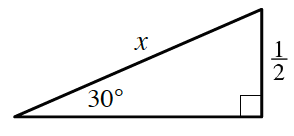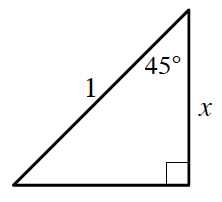### Home > INT3 > Chapter 9 > Lesson 9.1.2 > Problem9-25

9-25.

What is the length of the side labeled $x$? Write your answers in exact form.

1.Either use the special right triangle side length ratios or the Law of Sines.

$x = 1$

1.How can the Triangle Angle Sum Theorem help you find the missing angle?

Notice that this is a special right triangle with special side length ratios.